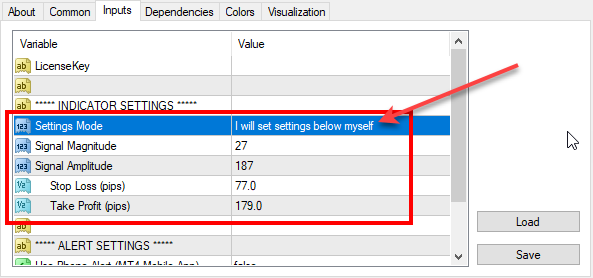# Re-optimized FxMagnetic indicator on EURJPY (2019-10-05)

We’ve reoptimized FxMagnetic indicator settings on EURJPY. Here are the latest parameters for all EURJPY timeframes that you should use for best MT4 indicator performance.To set parameters manually you need to switch the Settings Mode to: I will set settings below myself

Don’t have FxMagnetic indicator yet? You can buy FxMagnetic here.

## 1-minute chart:

Signal Magnitude = 159

Signal Amplitude = 44

Stop Loss (pips) = 17

Take Profit (pips) = 151

## 5-minute chart:

Signal Magnitude = 160

Signal Amplitude = 40

Stop Loss (pips) = 63

Take Profit (pips) = 129

## 15-minute chart:

Signal Magnitude = 10;

Signal Amplitude = 81;

Stop Loss (pips) = 71;

Take Profit (pips) = 159;

## 30-minute chart:

Signal Magnitude = 39;

Signal Amplitude = 27;

Stop Loss (pips) = 110;

Take Profit (pips) = 168;

## 1-hour chart:

Signal Magnitude = 51;

Signal Amplitude = 76;

Stop Loss (pips) = 67;

Take Profit (pips) = 169;

## 4-hour chart:

Signal Magnitude = 30;

Signal Amplitude = 56;

Stop Loss (pips) = 149;

Take Profit (pips) = 226;

## Daily chart:

Signal Magnitude = 89;

Signal Amplitude = 44;

Stop Loss (pips) = 318;

Take Profit (pips) = 514;

## Weekly chart:

Signal Magnitude = 10;

Ind0Param1 = 60;

Stop Loss (pips) = 350;

Take Profit (pips) = 2100;

## Monthly chart:

Signal Magnitude = 20;

Signal Amplitude = 40;

Stop Loss (pips) = 500;

Take Profit (pips) = 1600;

## FxMagnetic EURJPY v1.2 stats on 5th October, 2019

Below are the screenshots of FxMagnetic EURJPY stats from the previous 100 signals. This was taken on 2019-10-05 on the FxOpen Live ECN account. We re-optimized FxMagnetic EURJPY v1.2 on this broker so you’ll see the best signals with them.

Remember, past performance does not guarantee future results.

We do not know the future and none of the world’s trading algorithms know the future. All the stats above and trading signals are based on pure mathematical formulas and probability theory.